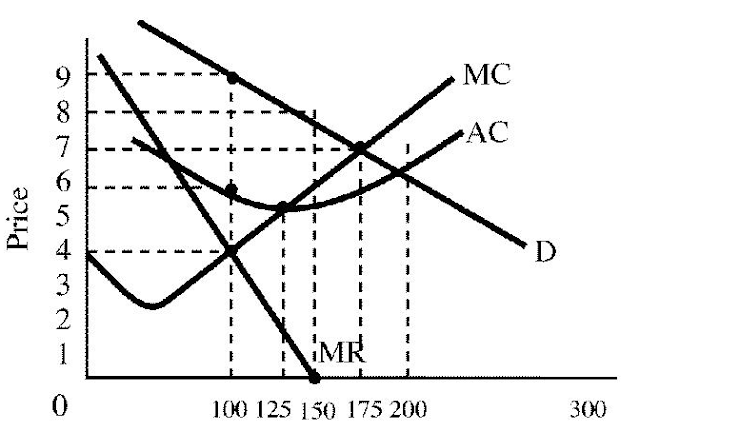A32-Marginal Revenue for an Imperfect Competitor
First name *
Last name *
SCHOOL email address *
Class period *
1. Explain why marginal revenue and price are NOT the same for imperfect competition. *
0 points
2. Why does the marginal revenue curve have a different slope (change) compared to demand? *
0 points
3. If the monopolist is charging one price to all consumers, why does it lower price? *
Look at the table
0 points
4. Refer to the graph below. Notice that the price points show \$1.50 changes. By how much does marginal revenue change for each change in price points? _____________ *
1 point
5. Explain your answer to number 4 above. How did you know to choose what you did? *
0 points
6. For a firm large enough to see the whole demand curve,marginal revenue is positive when the demand curve is price elastic.Marginal revenue becomes negative when the segment of the demand curve becomes price inelastic.Will a single-price monopoly ever operate on the inelastic portion of its demand curve? Why or why not? *
0 points
7. At which output, is total revenue maximized? *
HINT: It is the unit elastic point on the demand curve
1 point
8. Compare demand and marginal revenue in perfect competition and monopoly. What are the similarities? *
0 points
9. Compare demand and marginal revenue in perfect competition and monopoly. What are the differences? *
0 points